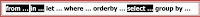## Monday, March 24, 2014

### LINQ

LINQ

1. LINQ stands for "Language Integrated Query".
2. This concept is introduced in .NET Framework 3.5.
3. This is a query writing technology.
4. This is most useful while working large amount of data in the live projects.
INTRODUCTION:
1. In relational database system, data is organized in the form of tables, on which you can write SQL queries to retrieve the required data according to the requirement in the application.
2. But you can‘t write a query on the non-database data, which in the form of objects in the application. There, you can write the queries using the new concept called "LINQ".
3. You can write queries on arrays, objects, databases and XML using LINQ.
4. Note: Before writing the LINQ queries, you should import the System.Linq namespace..
The following example shows a small demo on LINQ:
PROGRAM FOR LINQ TO ARRAYS
```
using System;
using System.Collections.Generic;
using System.Linq;
using System.Text;
namespace LINQtoArrayDemo
{
class Program
{
static void Main(string[] args)
{
//data source
int[] numbers = { 2, 12, 10, 5, 15, 4, 62 };
//ling query
IEnumerable result = from n in numbers where n <= 10 select n;
//output
foreach (var x in result)
Console.WriteLine(x);
}
}
}
```

In the above application, the array contains few numbers. After executing the query, you got only the numbers, which are less than 10. In this manner, you can execute the queries on data sets (after learning ADO.NET) also.

LINQ SYNTAX:THE ABOVE SYNTAX CONSISTS OF 7 CLAUSES.

1. from clause
2. in clause
3. let clause
4. where clause
5. orderby clause
6. select clause
7. group by clause
8. Mandatory clauses:
9. from clause
10. in clause
11. select clause
CLAUSE

Def of Clause: A part of the query.

UNDERSTANDING CLAUSES:
1. from clause: This is used to specify the iteration variable name. This acts as alias name for the data source.
2. in clause: This is used to specify the main data source for the query.
3. let clause (optional): This is used to declare a new identifier with a value, that is to be used during the query execution.
4. where clause (optional): This is most frequently used optional clause, using which you can specify the condition in the query.
5. orderby clause (optional): This is used to specify the sorting expression if required.
6. select clause: This is used to specify the object, which is required in the query results.
7. group by (optional): This is similar to ―group by‖ clause in SQL. This retrieves grouped data, based on a column.

Note: The result of a LINQ query should be assigned into a IEnumerable type variable. IEnumerable is an interface.

Library: System.Collections.Generic.IEnumerable
PROGRAM FOR LINQ TO OBJECTS
```Student.cs
using System;
using System.Collections.Generic;
using System.Linq;
using System.Text;
namespace LINQtoObjectsDemo
{
class Student
{
//fields
public int StudentID;
public string Name;
public string Course;
public int Marks;
//constructor
public Student(int StudentID, string Name, string Course, int Marks)
{
this.StudentID = StudentID;
this.Name = Name;
this.Course = Course;
this.Marks = Marks;
}
}
}

Program.cs
using System;
using System.Collections.Generic;
using System.Linq;
using System.Text;
namespace LINQtoObjectsDemo
{
class Program
{
static void Main(string[] args)
{
//data source
Student[] stu = { new Student(101, "Prakash", "MBA", 765),
new Student(102, "Pradeep", "MBA", 471),
new Student(103, "Pushpa", "Msc", 590),
new Student(104, "Purna", "MCA", 223),
new Student(105, "Purnima", "MCA", 450)};
//linq query with where clause
IEnumerable result1 = from s in stu where s.Course == "MCA" select s;                                                                                          Console.WriteLine("MCA Students:");
foreach (Student r in result1)
Console.WriteLine(r.StudentID + ", " + r.Name + ", " + r.Course + ", " + r.Marks);
//linq query with compound where clause
IEnumerable result2 = from s in stu where s.Name.EndsWith("a") && s.Marks>=400 &&                                                             s.Marks<=600 select s;
Console.WriteLine("\nStudents whose name ends with 'a', and marks is >=400 and <=600:");
foreach (Student r in result2)
Console.WriteLine(r.StudentID + ", " + r.Name + ", " + r.Course + ", " + r.Marks);

//linq query with let and where clauses
IEnumerable result3 = from s in stu let avg = s.Marks / 10 where avg < 35 select s;
Console.WriteLine("\nFailed Students:");
foreach (Student r in result3)
Console.WriteLine(r.StudentID + ", " + r.Name + ", " + r.Course + ", " + r.Marks);
//linq query with orderby clause
IEnumerable result4 = from s in stu orderby s.Marks select s;
Console.WriteLine("\nStudents (sort on marks):");
foreach (Student r in result4)
Console.WriteLine(r.StudentID + ", " + r.Name + ", " + r.Course + ", " + r.Marks);
//linq query with orderby clause (descending)
IEnumerable result5 = from s in stu orderby s.Marks descending select s;
Console.WriteLine("\nStudents (sort on marks - descending):");
foreach (Student r in result5)
Console.WriteLine(r.StudentID + ", " + r.Name + ", " + r.Course + ", " + r.Marks);
//linq query with group clause
IEnumerable> result6 = from s in stu group s by s.Course;
Console.WriteLine("\nStudents with grouping:");
foreach (IGrouping StuGrp in  result6)
{
Console.WriteLine(StuGrp.Key + ":");
foreach (Student r in StuGrp)
Console.WriteLine(" " + r.StudentID + ", " + r.Name + ", " + r.Course + ", " + r.Marks);
}